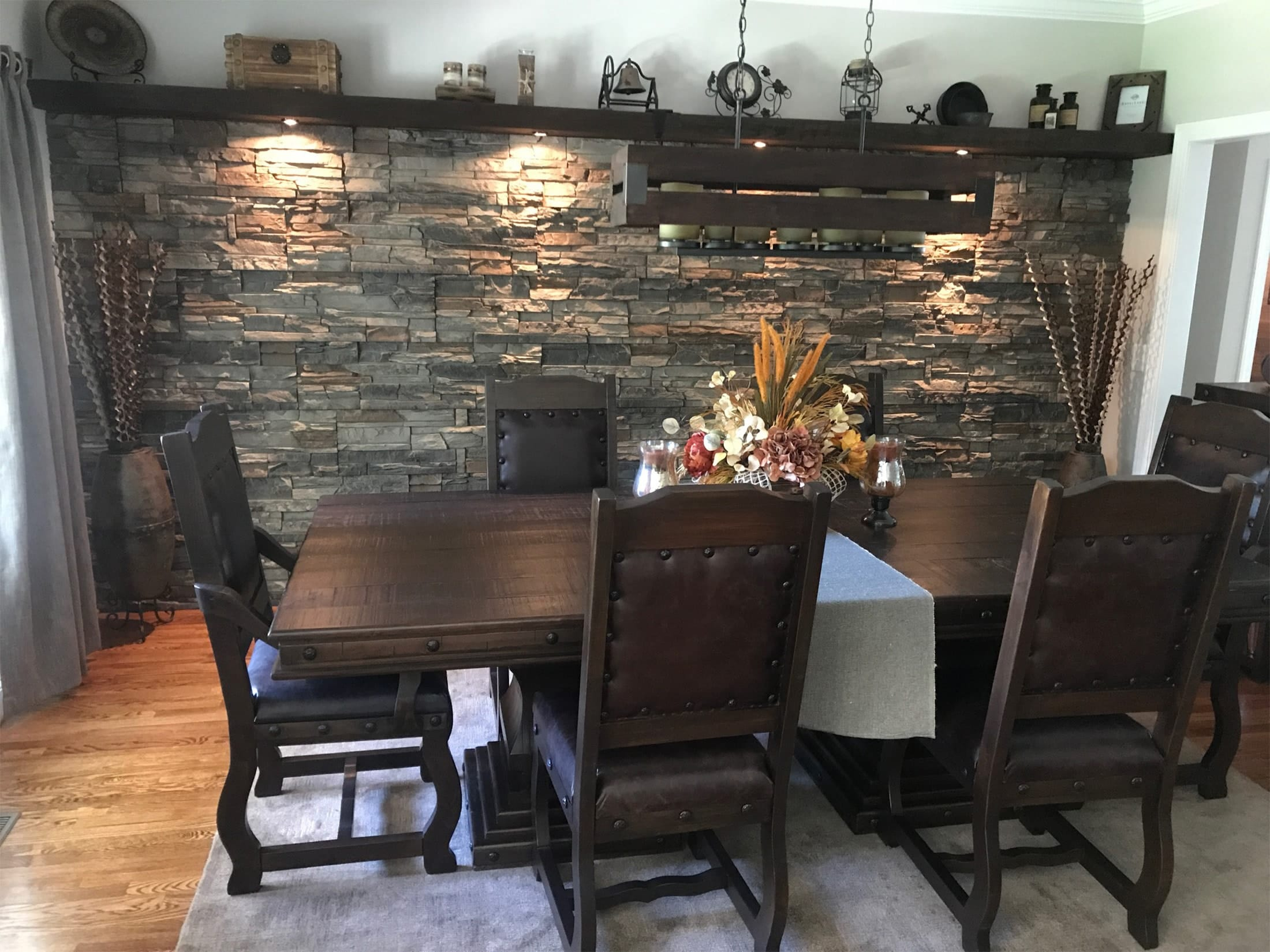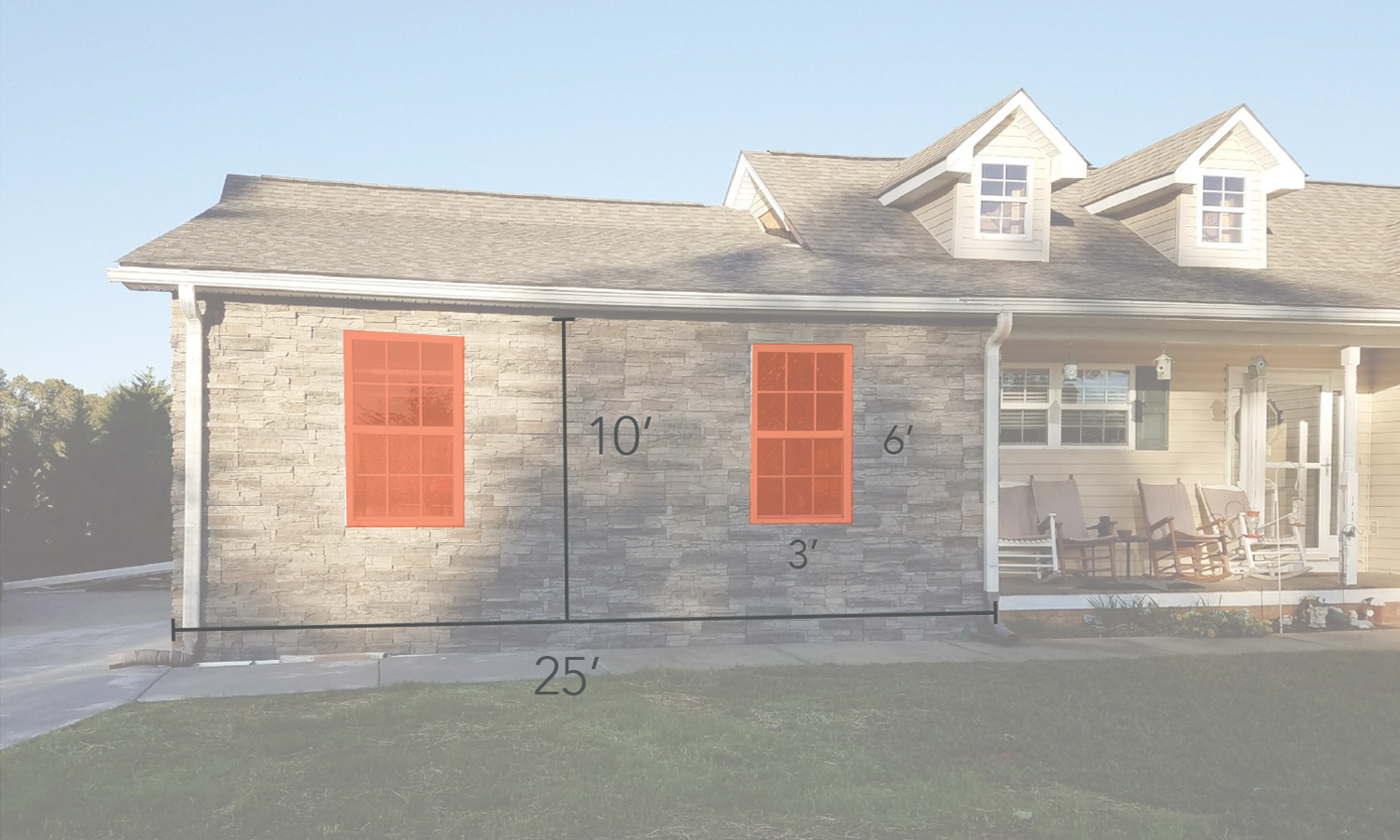Price My Project

# How to Measure

### Small Projects

Typically Interior

We recommend recording the width and height of your improvement area in inches. After you have the dimensions, you divide the width in inches by the coverage area in inches of the stacked stone or brick panel which will give you the number of panels you will need horizontally. Next, divide the height in inches by the coverage height of the panels. Finally, multiply the number needed horizontally by the number needed vertically.### Large Projects

Usually Exterior

Calculating the square footage of your install area is typically the best way to go when it comes to exterior projects. To find the square footage, measure the length and height of each wall in feet you plan to install GenStone on. Next, multiply the length by the height to determine the total square footage. If you are covering multiple walls, add the square footage of each wall together to find the total square footage. Lastly, you divide the square footage of your install area by the coverage area of the panel.### Ledgers and Trim

Inches vs Feet

The same rules apply when it comes to trim and ledgers, use inches if your installation area is small and feet if you are planning a larger scale project. Both the straight ledger and trim pieces are 3.50 linear feet (42″) long. Corner ledgers are recommended anywhere you plan to install a corner and want to continue the ledger into the next wall and you can subtract one straight ledger for every two corner ledgers needed.

### Ledgers

Ledgers are most commonly used for wainscot projects. To determine how many stacked stone or brick ledgers you need, measure the length of your wall in feet or inches. Next, divide your measurement by 42 if using inches or 3.50 if you recorded your dimensions in feet.

### Trim

Trim is useful around obstacles like electrical boxes, hose bibs and other common voids. to determine the quantity needed, divide your measurement by 42 if using inches or 3.50 if you recorded your dimensions in feet.

### Accounting for Voids

The Last Step

The final step in measuring for your project is to account for voids such as doors and windows that are directly in your installation area. Simply subtract the dimensions of these voids from your final count of inches or square footage to arrive at the correct number of panels you will need to complete your project.

In this example, you would subtract 36 square feet from the installation area to account for the two windows.Send this to a friend